# Search by Topic

#### Resources tagged with Angles - points, lines and parallel lines similar to Flight Path:

Filter by: Content type:
Age range:
Challenge level:

### There are 27 results

Broad Topics > Angles, Polygons, and Geometrical Proof > Angles - points, lines and parallel lines### Flight Path

##### Age 16 to 18 Challenge Level:

Use simple trigonometry to calculate the distance along the flight path from London to Sydney.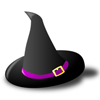### Witch's Hat

##### Age 11 to 16 Challenge Level:

What shapes should Elly cut out to make a witch's hat? How can she make a taller hat?### Orbiting Billiard Balls

##### Age 14 to 16 Challenge Level:

What angle is needed for a ball to do a circuit of the billiard table and then pass through its original position?### Pegboard Quads

##### Age 14 to 16 Challenge Level:

Make five different quadrilaterals on a nine-point pegboard, without using the centre peg. Work out the angles in each quadrilateral you make. Now, what other relationships you can see?### Lunar Angles

##### Age 16 to 18 Challenge Level:

What is the sum of the angles of a triangle whose sides are circular arcs on a flat surface? What if the triangle is on the surface of a sphere?### Octa-flower

##### Age 16 to 18 Challenge Level:

Join some regular octahedra, face touching face and one vertex of each meeting at a point. How many octahedra can you fit around this point?### Spirostars

##### Age 16 to 18 Challenge Level:

A spiropath is a sequence of connected line segments end to end taking different directions. The same spiropath is iterated. When does it cycle and when does it go on indefinitely?### LOGO Challenge 1 - Star Square

##### Age 7 to 16 Challenge Level:

Can you use LOGO to create this star pattern made from squares. Only basic LOGO knowledge needed.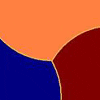### Three Tears

##### Age 14 to 16 Challenge Level:

Construct this design using only compasses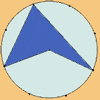### Angle Measurement: an Opportunity for Equity

##### Age 11 to 16

Suggestions for worthwhile mathematical activity on the subject of angle measurement for all pupils.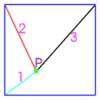### Square World

##### Age 16 to 18 Challenge Level:

P is a point inside a square ABCD such that PA= 1, PB = 2 and PC = 3. How big is angle APB ?### Flexi Quads

##### Age 16 to 18 Challenge Level:

A quadrilateral changes shape with the edge lengths constant. Show the scalar product of the diagonals is constant. If the diagonals are perpendicular in one position are they always perpendicular?### Making Sixty

##### Age 14 to 16 Challenge Level:

Why does this fold create an angle of sixty degrees?### Same Length

##### Age 11 to 16 Challenge Level:

Construct two equilateral triangles on a straight line. There are two lengths that look the same - can you prove it?### Semi-regular Tessellations

##### Age 11 to 16 Challenge Level:

Semi-regular tessellations combine two or more different regular polygons to fill the plane. Can you find all the semi-regular tessellations?### Angle Trisection

##### Age 14 to 16 Challenge Level:

It is impossible to trisect an angle using only ruler and compasses but it can be done using a carpenter's square.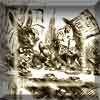### A Problem of Time

##### Age 14 to 16 Challenge Level:

Consider a watch face which has identical hands and identical marks for the hours. It is opposite to a mirror. When is the time as read direct and in the mirror exactly the same between 6 and 7?### Interacting with the Geometry of the Circle

##### Age 5 to 16

Jennifer Piggott and Charlie Gilderdale describe a free interactive circular geoboard environment that can lead learners to pose mathematical questions.### LOGO Challenge 7 - More Stars and Squares

##### Age 11 to 16 Challenge Level:

Can you use LOGO to create a systematic reproduction of a basic design? An introduction to variables in a familiar setting.### LOGO Challenge 8 - Rhombi

##### Age 7 to 16 Challenge Level:

Explore patterns based on a rhombus. How can you enlarge the pattern - or explode it?### Parallel Universe

##### Age 14 to 16 Challenge Level:

An equilateral triangle is constructed on BC. A line QD is drawn, where Q is the midpoint of AC. Prove that AB // QD.### Similarly So

##### Age 14 to 16 Challenge Level:

ABCD is a square. P is the midpoint of AB and is joined to C. A line from D perpendicular to PC meets the line at the point Q. Prove AQ = AD.### Arrowhead

##### Age 14 to 16 Challenge Level:

The points P, Q, R and S are the midpoints of the edges of a non-convex quadrilateral.What do you notice about the quadrilateral PQRS and its area?### Hand Swap

##### Age 14 to 16 Challenge Level:

My train left London between 6 a.m. and 7 a.m. and arrived in Paris between 9 a.m. and 10 a.m. At the start and end of the journey the hands on my watch were in exactly the same positions but the. . . .### Quad in Quad

##### Age 14 to 16 Challenge Level:

Join the midpoints of a quadrilateral to get a new quadrilateral. What is special about it?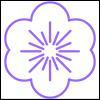### Flower

##### Age 16 to 18 Challenge Level:

Six circles around a central circle make a flower. Watch the flower as you change the radii in this circle packing. Prove that with the given ratios of the radii the petals touch and fit perfectly.### Robotic Rotations

##### Age 11 to 16 Challenge Level:

How did the the rotation robot make these patterns?